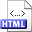Sorting multisets stably in minimum space Authors: Jyrki Katajainen and Tomi Pasanen Published in: Proceedings of the 3rd Scandinavian Workshop on Algorithm Theory, Lecture Notes in Computer Science 621, Springer-Verlag (1992), 410-421 DOI: 10.1007/3-540-55706-7_37 Copyright: © Springer-Verlag Abstract: In a decision tree model, Ω(nlog2n − ∑i=1m nilog2ni+n) is known to be a lower bound for sorting a multiset of size n containing m distinct elements, where the ith distinct element appears ni times. We present a minimum space algorithm that sorts stably a multiset in asymptotically optimal worst-case time. A Quicksort type approach is used, where at each recursive step the median is chosen as the partitioning element. To obtain a stable minimum space implemention, we develop linear-time in-place algorithms for the following problems, which have interest of their own: Stable unpartitioning: Assume that an n-element array A is stably partitioned into two subarrays A0 and A1. The problem is to recover A from its constituents A0 and A1. The information available is the partitioning element used and a bit array of size n indicating whether an element of A0 or A1 was originally in the corresponding position of A. Stable selection: The task is to find the kth smallest element in a multiset of n elements such that the relative order of identical elements is retained. Related:HTML (Journal article) BibLATEX: ```@inproceedings{KP1992dC, author = {Jyrki Katajainen and Tomi Pasanen}, title = {Sorting multisets stably in minimum space}, booktitle = {Proceedings of the 3rd Scandinavian Workshop on Algorithm Theory}, series = {Lecture Notes in Computer Science}, volume = {621}, publisher = {Springer-Verlag}, year = {1992}, pages = {410--421}, } ```